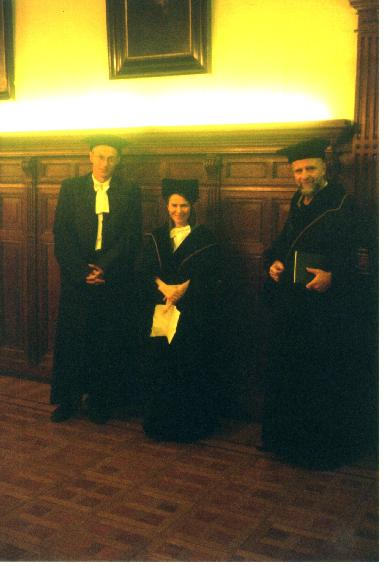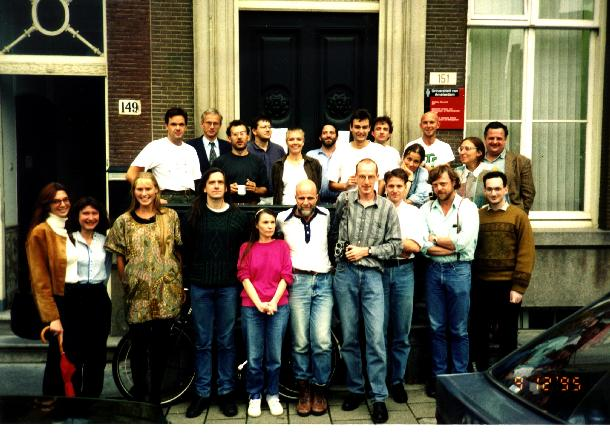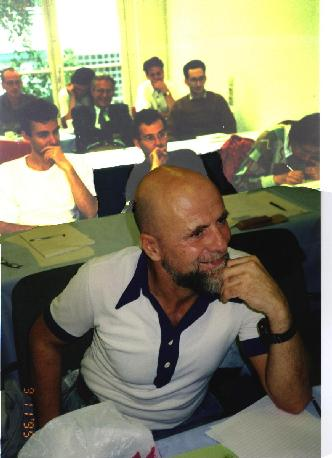# Logical Analysis of Special Relativity Theory

## Hajnal Andréka, Judit X. Madarász, and István Németi

Alfréd Rényi Mathematical Institute
P.O.Box 127
Budapest
H-1364 Hungary

### Abstract

Here we outline an approach to a logical analysis of relativity theory conducted purely in first order logic (for methodological reasons). Here we concentrate on special relativity, but in a more extensive work referred to here as (AMNSS 1998), some steps are made in the direction of generalizing the present approach towards general relativity. In (AMNSS 1998) we build up variants of relativity theory as ``competing'' axiom systems formalized in first order logic. The reason for having several versions for the theory, i.e. several axiom systems, is that this way we can study the consequences of the various axioms, enabling us to find out which axiom is responsible for some interesting or ``exotic'' prediction of relativity theory. Among others, this enables us to refine the conceptual analysis in Friedman's and Rindler's books, or compare the Reichenbach-Grunbaum approach to relativity with the standard one.

After having formalized relativity in first order logic, we use the well developed machinery of first order logic for studying properties of the theory (e.g. the number of non-elementarily equivalent models, or its relationships with Goedel's incompleteness theorems, independence issues etc).

In the present paper, first we recall one of our axiom systems ``Specrel'' for relativity from (AMNSS 1998). Then we present some of the typically logical investigations of Specrel, e.g. independence of the axioms, consistency properties of models. We also discuss the intuitive contents (and consequences) of some of the axioms. We also present a little bit of the conceptual analysis part of (AMNSS 1998) using the example of Specrel and ``FTL observers''. Finally, we study how much Goedel's incompleteness theorems apply to Specrel: Specrel is undecidable, and it admits natural extensions some of which are decidable, while to others the full strength of both of Goedel's incompleteness theorems applies. E.g. there is an extension Specrel+ in which its own consistency is formalizable (and is neither provable nor refutable). Specrel+ is hereditarily undecidable.

We deliberately try to keep the number of axioms in Specrel small, and their intuitive contents simple, transparent, and tangible from the logical point of view.

Dvi-file
PS-File
PDF-File
Bibtex Entry